# B. Generating a Distribution for the Droplet Radius

The random distribution for the droplet radius for the ESD spray pyrolysis model in (6.1) is derived in this section. The volume fraction is evenly distributed along the droplets whose radii range from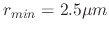to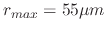. Therefore, the first step is to relate the radius distribution linearly to a value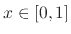so that as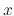goes from 0 to 1,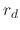goes from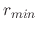to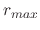:(249)

Next, the assertion is made thatrepresents the evenly distributed volume number fraction, or normalized volume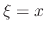. Using the equation for the volume of a sphere, the relationship between volume and radius is established as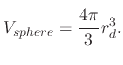(250)

Therefore, when the volume is evenly distributed, the effect on the radius will be. Initially, it might be counter-intuitive to note the inverse relationship since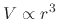. However, when a volume of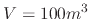is distributed for a radius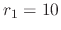m, then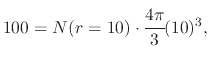(251)

where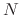is the number fraction, resulting in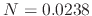. When the same volume is distributed for droplets of a radius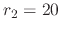m, then the calculation above leads to the number fraction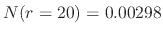, which is 8 times less, or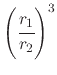times less.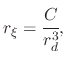(252)

where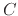is a normalization constant which must be found,is the randomly distributed radius, andis the radius relatingandto an even volume distribution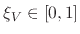from (B.1)(253)

Inverting (B.5) to solve for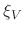allows to find the CPD function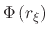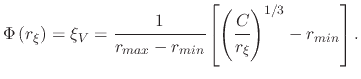(254)

The derivative of (B.6) gives the PDF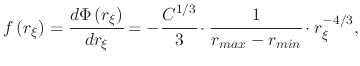(255)

where it can be noted that the lastterm from (B.6) has disappeared. The only non-constant term in (B.7) is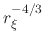, therefore a replacement constant, which will be the new normalization constant is introduced for simplicity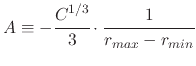(256)

and (B.7) can be rewritten to(257)

Using the PDF from (B.9), we can now proceed to find the normalized distribution, but first the normalization constant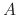must be found by integrating (B.9) with respect tofromtoand equating the integral to 1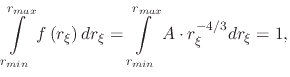(258)

which can be solved to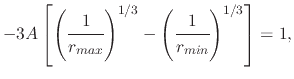(259)

giving the normalization constant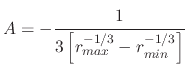(260)

and the normalized PDF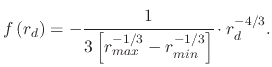(261)

Now one can integrate the normalized PDF fromtoto find the CPD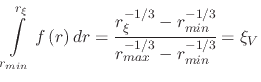(262)

and invert the CPD to find the quantile function and solve for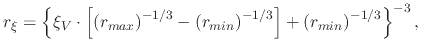(263)

which gives the equation for the radius distributionbetweenandwhen the volume number fractionis evenly distributed and.

L. Filipovic: Topography Simulation of Novel Processing Techniques